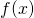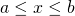# Evaluating Integrals with ModulusMany students seem to struggle when they see an integral with modulus as they do not know where to begin with. The first thing they should note is that, we cannot evaluate an integral with modulus directly, that means, we must remove (address) the modulus first.

So let’s see how we should approach such questions, considering, we must first know what range of values offor whichis negative, in this case, let us assume thatfor, where.

ThenWe break the integral up into two parts, adding a negative sign to the integral part for which. Students can relate this to reflectingabout the x-axis to make it a positive area.

Students may want to reference this recent ACJC Prelim 2015 Question to see if they can do it.

Not readable? Change text.